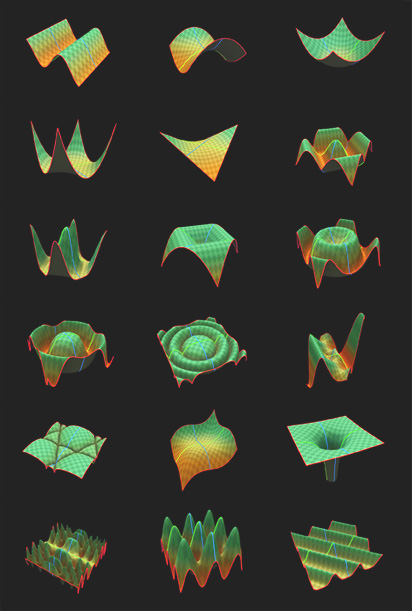# Graphing in ICE

Visualize the graph of a function is not easy (at least for me), without plotting the function.
It can be really hard to understand what y = x^2 * z^2 looks like.
So I obviously did an ICE compound with these most common graphs.
I couldn’t stop doing functions and playing with it, so I ended up with this list, all in the same compound:
x*z
x^2 + z^2
x^2 – z^2
x^2 * z^2
x^3 + z^3
sin(x)
cos(x) + cos(z) ^ 2
cos(x^2 + z^2)
sin(x^2 + z^2)
-1 / (x^2 + z^2)
|cos(x) + cos(z)| ^ (1/2)
cos(x) + cos(z)
| cos(x^2+z^2) | ^ (1/2)
|cos(x+z)| ^ (10/2)
cos( |x| + |z| ) * ( x + z )
cos( |x| + |z|) * (|x| + |z| )
cos( |x| + |z| )
|sin(x) * ( sin(x) + cos(z) )| ^ 0.2
sin(x^2) * cos(z^2)

Some are very common, others are not. It’s cool how many crazy patters you can get using these functions.

Hopefully this will work as a “educational” resource, so you can see what these functions look when applied to a 3d shape and understand how Sin, Cos, Absolute values, etc, can affect the function.

Here is what these equations look like when applied to a grid:What’s even more cool in ICE is that after I had all the functions done, I could apply this as a force, a weightmap… basically anything that reads a 3d vector or a scalar.

Tags: , , , , ,